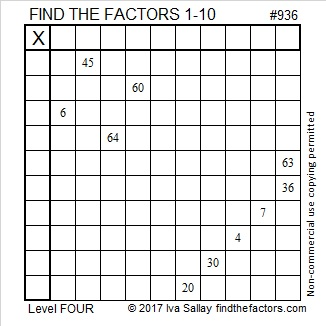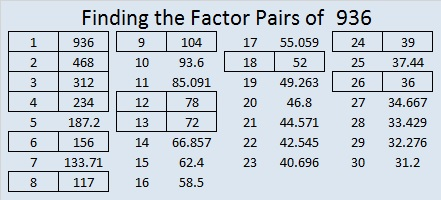# 936 and Level 4

Will this puzzle be smooth sailing for you? You won’t know until you give it a try!Print the puzzles or type the solution on this excel file: 10-factors-932-941

936 is the 12th pentagonal pyramidal number because 12²(12 + 1)/2 = 936.

936 is the sum of the twelve prime numbers from 53 to 103.

30² + 6² = 936, so 936 is the hypotenuse of a Pythagorean triple:
360-864-936 which is (5-12-13) times 72, and
can be calculated from 2(30)(6), 30² – 6², 30² + 6²

936 is palindrome 12221 in BASE 5 because 1(5⁴) + 2(5³) + 2(5²) + 2(5¹) + 1(5º) = 936
and palindrome QQ in BASE 35 (Q is 26 base 10), because 26(35) + 26(1) = 26(36) = 936
It is also Q0 in BASE 36 because 26(36) + 0(1) = 936

• 936 is a composite number.
• Prime factorization: 936 = 2 × 2 × 2 × 3 × 3 × 13, which can be written 936 = 2³ × 3² × 13
• The exponents in the prime factorization are 3, 2 and 1. Adding one to each and multiplying we get (3 + 1)(2 + 1)(1 + 1) = 4 × 3 × 2 = 24. Therefore 936 has exactly 24 factors.
• Factors of 936: 1, 2, 3, 4, 6, 8, 9, 12, 13, 18, 24, 26, 36, 39, 52, 72, 78, 104, 117, 156, 234, 312, 468, 936
• Factor pairs: 936 = 1 × 936, 2 × 468, 3 × 312, 4 × 234, 6 × 156, 8 × 117, 9 × 104, 12 × 78, 13 × 72, 18 × 52, 24 × 39¸ or 26 × 36
• Taking the factor pair with the largest square number factor, we get √936 = (√36)(√26) = 6√26 ≈ 30.594117.This site uses Akismet to reduce spam. Learn how your comment data is processed.